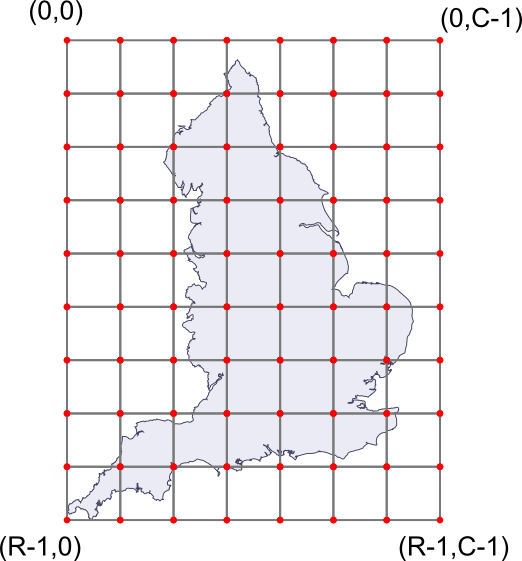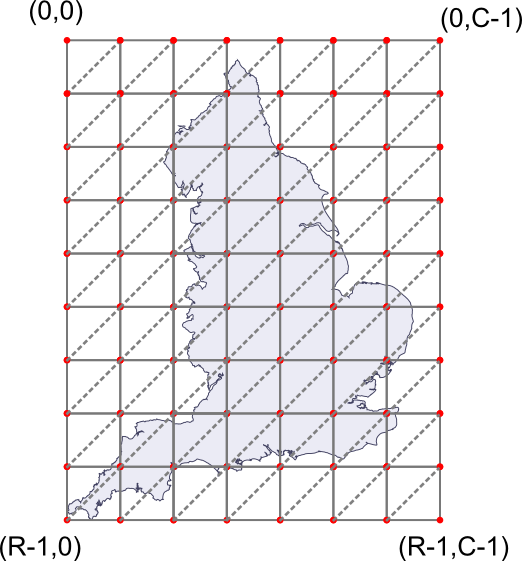## Measuring Volume of Land

• 문제 ID
• 시간 제한
• 메모리 제한
• 제출 횟수
• 정답 횟수 (비율)
• 출처
• 분류

#### 문제

Recently, Frograms Inc. acquired a calm and beautiful uninhabited island in the Pacific Ocean to set up their new headquarter office. Before embarking on the construction, we want to come up with a good disaster plan. Luckily, the new office is architected to resist catastrophes such as hurricane, earthquake, and nuclear plant meltdowns. But there is one thing we haven't considered yet; the global warming.

Experts agree that in the future, as the average temperature of Earth rises, the ice sheets in Antartica and Greenland will melt and the global sea level will rise up to 6 meters. We want to avoid the situation in which our office gets underwater.

Therefore, you are given with the following task: given a heightmap of the island, calculate the volume of the land mass above sea level after the sea level rises by L meters, approximated from using triangulated terrains from a heightmap. If you don't know what heightmaps or triangulated terrains are, don't worry and read on.To measure the terrain landscape, a commercial satelite will scan a rectangular area of R-1 meters by C-1 meters. (You can be sure that this area will completely enclose the island.) The area is then divided into an uniform grid with RxC grid points, and the satelite will measure the elevation above sea level (in centimeters) at every grid point. The above figure gives such an example; the elevation will be measured for every red point in the grid. The resulting 2D array is called a heightmap, and this will be given as your input.

Given a heightmap, there are multiple ways to approximate the actual shape of the terrain. One obvious way is to assume that each point in a heightmap correspond to a 1x1 meter square with the given elevation (if you know what it is, think Minecraft) -- but those approximations are too crude.

Another way that is still simple, but gives a better precision is approximating the surface as a set of triangles in the 3D space. We can do it as follows: let's take all the squares in the grid, and cut them diagonally to make two congruent triangles. For any square with four corners at (r,c), (r+1,c), (r,c+1) and (r+1,c+1), we cut them through the line passing (r+1,c) and (r,c+1). See below for an example.After that, we can shift each grid point in the 3D space vertically to match its measured height. Now, each triangle connects three points in the 3D space. This is how we approximate the shape of the terrain.

#### 입력

The input consists of T test cases. The number of test cases T is given in the first line of the input.

The first line of each test case will contain three integers R, C (3 <= R, C <= 100) and L (0 <= L <= 216 - 1). The following R lines will contain the heightmap. Each of those lines will contain C nonnegative integers Hr, c (0 <= Hr, c <= 216 - 1), representing the elevation of the point above sea level, in centimeters.

Since the island is on the sea, the measurements at the border of the heightmap are always zero.

#### 출력

Print exactly one line for each test case. The line should contain the volume of the land in unit of cubic meters(m3), assuming the sea level has risen by L meters (including lakes). Your result should be accurate to within a relative or absolute error of 10-8.

#### 예제 입력

2
3 3 0
0 0 0
0 100 0
0 0 0
4 5 50
0 0 0 0 0
0 100 50 50 0
0 50 100 50 0
0 0 0 0 0

#### 예제 출력

1.00000000000
0.58333333333

#### 노트

• 출제: Being
• 디스크립션 작성: JongMan
• 검수: astein, altertain
• 그림: JongMan